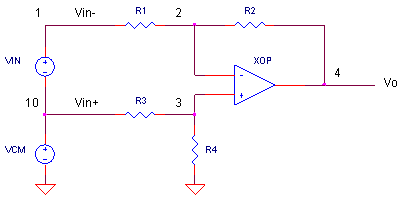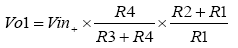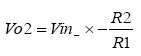eCircuit  Center

### Op Amp Differential Amplifier

CIRCUITThe differential amplifier has a unique feature that many circuits don’t have - two inputs. This circuit amplifies the difference between its input terminals. Many circuits that have one input, actually have another input – the ground potential.  But, in cases where a source (like a sensor signal of 10mV) can have both its terminals biased at ~5V above ground (like a sensor bridge), you need to amplify the difference between the terminals and reject the 5V bias.

What about noise that adds an unwanted voltage equally to both terminals of a sensor? The differential amp rejects the noise common to both signals and rescues the signal difference between the input terminals.

VOLTAGE GAIN

How does a differential amp amplify only the difference between the two inputs? First, let's write the output due to each input. Driving the positive input Vin+ and setting the negative input Vin- = 0V, you get Vo1Alternately, driving Vin- and setting Vin+ = 0V, you get Vo2To put it all total together, add  the two outputs to get Vo = Vo1 + Vo2.It's not a differential amp just yet! However, by choosing ratios R2/R1 = R4/R3 you make the following equality:  R4/(R3+R4)=R2/(R1+R2). Then substitute this in the equation above and simplify to getOnly the difference gets amplified. What about signals common (or equal) at both inputs
Vin+ and Vin-? The equation tells you the output Vo should be zero! This Common Mode Rejection (CMR) is useful but not perfect! It depends on the op amp device itself and matching of the resistor values (more below).

DIFF AMP TEST

Suppose you have a 1Vpeak sine wave that needs to amplified to 5V. Unfortunately, this signal rides on top of a 5V interfering signal. The SPICE circuit applies a difference signal VIN (1V @ 10kHz) and a common-mode signal VCM (5V @ 1kHz) to the amplifier’s inputs.

CIRCUIT ANALYSIS   With R1=R2=10k, the gain of the circuit is 1. Run a simulation and watch the total input V(1) and output V(4).  Did the circuit amplify VIN (10kHz) and reject VCM (1 kHz)?

HANDS-ON DESIGN  You can easily design a circuit for a different voltage gain. (For example, choosing R1 = R4 = 50k creates a gain of 50/10 = 5 V/V.) Run a simulation and view your new output.

COMMON MODE REJECTION TEST

CIRCUIT ANALYSIS   One way to test CMR is to kill voltage source VIN and see how much of the common-mode signal VCM gets through to the output. Set VIN to 0V by the statement

VIN     1     10     SIN(0  0  10KHZ)

Run a simulation and see how much VCM squeaks through. To get a closer view of the output, plot V(4) only.

RESISTOR MATCHING

The ability of the circuit to reject common signals depends on how well the resistor ratios are matched. How much mismatch does it take to degrade the CMR of a differential amp?

CIRCUIT ANALYSIS   Suppose just one of the resistors of the resistors is off by only 0.1%.  Change any one of resistors R1-R4 to 10.01 k or 9.999 k. Try the circuit to see how much the output increases from VCM due to resistor mismatching. Increase the mismatch to 1% by changing any resitor to 10.1 k or 9.99 k. How much common mode error gets though now?

SPICE FILE

Download the file or copy this netlist into a text file with the *.cir extention.

```OPDIF.CIR - DIFFERENTIAL AMPLIFIER
*
VIN	1	10	SIN(0	1	10KHZ)
VCM	10	0	SIN(0	5	1KHZ)
*
R1	1	2	10K
R2	2	4	10K
R3	10	3	10K
R4	3	0	10K
XOP	3 2	4	OPAMP1
*
* OPAMP MACRO MODEL, SINGLE-POLE
* connections:      non-inverting input
*                   |   inverting input
*                   |   |   output
*                   |   |   |
.SUBCKT OPAMP1	    1   2   6
* INPUT IMPEDANCE
RIN	1	2	10MEG
* DC GAIN (100K) AND POLE 1 (100HZ)
* GBWP = 10MHz
EGAIN	3 0	1 2	100K
RP1	3	4	1K
CP1	4	0	1.5915UF
* OUTPUT BUFFER AND RESISTANCE
EBUFFER	5 0	4 0	1
ROUT	5	6	10
.ENDS
*
.TRAN 	0.01MS  1MS
.PROBE
.END```

top